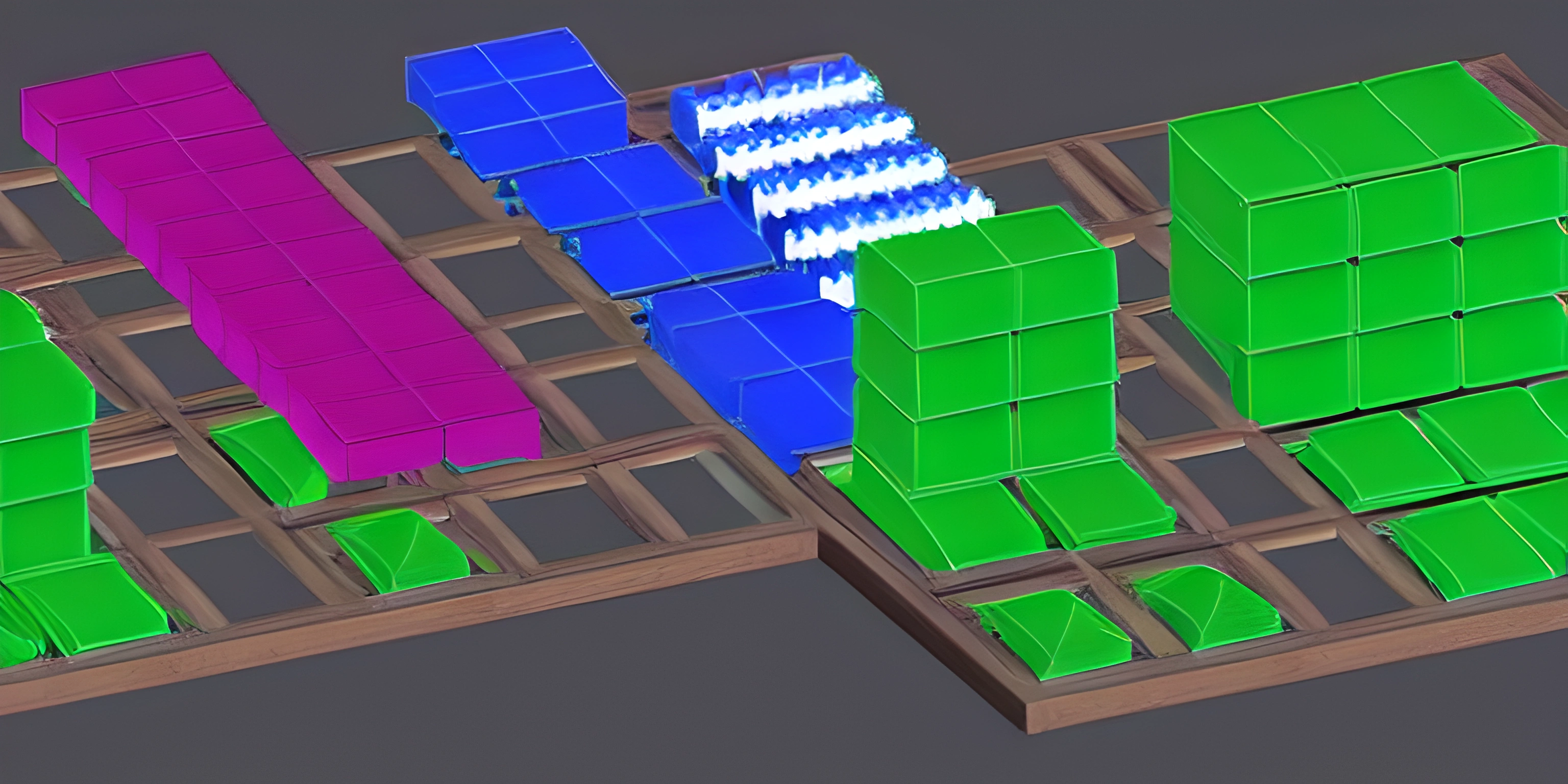# Array Sorting TechniquesNote: this page has been created with the use of AI. Please take caution, and note that the content of this page does not necessarily reflect the opinion of Cratecode.

Sorting is a fundamental operation in programming, and it's something that we often need to do when working with data. Arrays are a common data structure, and sorting them can be done in many different ways. In this article, we'll dive into various array sorting techniques, explore their advantages and disadvantages, and see examples in different programming languages.

## Bubble Sort

Bubble sort is one of the simplest sorting algorithms. It works by repeatedly stepping through the array, comparing each pair of adjacent elements and swapping them if they are in the wrong order. Bubble sort's main advantage is its simplicity, which makes it easy to understand and implement. However, its performance is generally poor, so it's not recommended for large datasets.

Here's an example of bubble sort in Python:

``````def bubble_sort(arr):
n = len(arr)
for i in range(n):
for j in range(0, n - i - 1):
if arr[j] > arr[j + 1]:
arr[j], arr[j + 1] = arr[j + 1], arr[j]

example_array = [64, 34, 25, 12, 22, 11, 90]
bubble_sort(example_array)
print("Sorted array is:", example_array)``````

## Selection Sort

Selection sort is another simple sorting algorithm. It works by dividing the input array into two parts: the sorted part, which starts empty, and the unsorted part. The algorithm repeatedly selects the smallest (or largest) element from the unsorted part and moves it to the end of the sorted part. Like bubble sort, selection sort's main advantage is its simplicity, but it's not efficient for large datasets.

Here's an example of selection sort in JavaScript:

``````function selectionSort(arr) {
const n = arr.length;
for (let i = 0; i < n - 1; i++) {
let minIndex = i;
for (let j = i + 1; j < n; j++) {
if (arr[j] < arr[minIndex]) {
minIndex = j;
}
}
if (minIndex !== i) {
[arr[i], arr[minIndex]] = [arr[minIndex], arr[i]];
}
}
}

const exampleArray = [64, 34, 25, 12, 22, 11, 90];
selectionSort(exampleArray);
console.log("Sorted array is:", exampleArray);``````

## Quick Sort

Quick sort is a more advanced sorting algorithm that is often faster in practice than bubble sort and selection sort. It works by choosing a "pivot" element from the array and partitioning the other elements into two groups – those less than the pivot and those greater than the pivot. The algorithm then recursively sorts the two groups. Quick sort's main advantage is its performance, but it can be more challenging to understand and implement.

Here's an example of quick sort in Ruby:

``````def quick_sort(arr)
return arr if arr.length <= 1

pivot = arr.delete_at(rand(arr.length))
less, greater = arr.partition { |e| e < pivot }

quick_sort(less) + [pivot] + quick_sort(greater)
end

example_array = [64, 34, 25, 12, 22, 11, 90]
sorted_array = quick_sort(example_array)
puts "Sorted array is: #{sorted_array.inspect}"``````

## Merge Sort

Merge sort is another efficient sorting algorithm, which is based on the divide-and-conquer approach. It works by dividing the array into two equal halves, recursively sorting each half, and then merging the two sorted halves back together. Merge sort's main advantage is its performance and stability, but it can be more challenging to understand and implement than simpler algorithms.

Here's an example of merge sort in Java:

``````public static void mergeSort(int[] arr, int left, int right) {
if (left < right) {
int mid = (left + right) / 2;
mergeSort(arr, left, mid);
mergeSort(arr, mid + 1, right);
merge(arr, left, mid, right);
}
}

public static void merge(int[] arr, int left, int mid, int right) {
int[] temp = new int[right - left + 1];
int i = left, j = mid + 1, k = 0;

while (i <= mid && j <= right) {
if (arr[i] <= arr[j]) {
temp[k++] = arr[i++];
} else {
temp[k++] = arr[j++];
}
}

while (i <= mid) {
temp[k++] = arr[i++];
}

while (j <= right) {
temp[k++] = arr[j++];
}

for (int t = 0; t < temp.length; t++) {
arr[left + t] = temp[t];
}
}

public static void main(String[] args) {
int[] exampleArray = {64, 34, 25, 12, 22, 11, 90};
mergeSort(exampleArray, 0, exampleArray.length - 1);
System.out.println("Sorted array is: " + Arrays.toString(exampleArray));
}``````

These are just a few examples of array sorting techniques. The best method to use depends on the specific requirements of your program and the size of the dataset you need to sort. Remember that understanding how these algorithms work, and when to use them, is essential for any programmer. So, keep exploring and deepening your knowledge in this area!